# python 3 之 Argparse 模块简介【实现解析命令行读取参数】

【摘要】今天接触了一个新知识，叫做 argparse 模块，是一个在当前实际工作中非常有用的 python 模块，所以赶紧写了篇文章记录一下，也分享给大家，能够更好的在实际工作中使用。

## （一）argparse 模块简介

1）argparse 模块是 python 的一个命令行解析包，可以十分方便的对 python 文件进行命令行读写

2）argparse 模块是 python 中自带的模块，不需要再安装

3）使用时先导入模块，import argparse

## （二）一般方法，利用 sys.argv

1. `import sys`
2. `print("Input argument is %s" %(sys.argv))`

1. `>python .demo.py how are you 123`
2. `Input argument is ['.\demo.py', 'how', 'are', 'you', '123']`

## （三）argparse 模块使用方法

1. `import argparse`
2. ` `
3. `if __name__ == '__main__':`
4. ` `
5. `    # 创建命令行解析器句柄`
6. `    parser = argparse.ArgumentParser(description='自定义描述信息，本脚本执行功能为：演示 argparse 模块')`
7. `  `
8. `    # 没有横杆开头的是必填参数，并且按照输入顺序进行设定`
9. `    parser.add_argument('positionArg',help='定义必选参数 positionArg（help 属性为提示信息）')`
10. `  `
11. `    # --verbosity1：两个横线 -- 开头，指的是变量名或者称为标签`
12. `    # -v1：一个横线 - 开通，指的是变量的别名或者标签别名`
13. `    parser.add_argument('--verbosity1', '-v1', help='定义可选参数 verbosity1') `
14. `   `
15. `    # 定义可选参数 state`
16. `    # 设定 action='store_true' 表示该选项不需要接收参数`
17. `    # 若不设 action，则默认是需要接收参数的，否则会报错`
18. `    parser.add_argument(`
19. `        '--state', `
20. `        '-s', `
21. `        action='store_true', `
22. `        help='若有 "-s" ，则 state = Ture,否则 state = False'`
23. `    )`
24. `     `
25. `    # 执行参数的类型`
26. `    parser.add_argument(`
27. `        '-int',`
28. `        '-i', `
29. `        type=int, `
30. `        help='指定参数类型（默认是 str）'`
31. `    )`
32. ` `
33. `    parser.add_argument(`
34. `        '-c', `
35. `        help='设置参数的可选值，即输入值必须为 choices 列表中的某个值，若未设置则默认值为 None',`
36. `        choices=['one', 'two', 'three', 'four'],`
37. `    )`
38. ` `
39. `    parser.add_argument(`
40. `        '--default',`
41. `        '-d', `
42. `        help='default 可以设置默认值',`
43. `        choices=['one', 'two', 'three', 'four'],`
44. `        default = 'one',`
45. `    )`
46. `  `
47. `    # 参数是否必填，使用 required 属性`
48. `    parser.add_argument(`
49. `        '--required',`
50. `        '-r', `
51. `        help='required 的值为：True 或者 False',`
52. `        required=True,`
53. `    )`
54. `  `
55. `    # 最后：获取、解析参数的值`
56. `    args = parser.parse_args()  # 返回一个命名空间`
57. `    print(args)`
58. `    params = vars(args)  # 返回字典`
59. `    print(params,type(params))`

1. `> python .demo2.py start -v1 10 -s -r helloworld`
2. `Namespace(c=None, default='one', int=None, positionArg='start', required='helloworld', state=True, verbosity1='10')`
3. `{'positionArg': 'start', 'verbosity1': '10', 'state': True, 'int': None, 'c': None, 'default': 'one', 'required': 'helloworld'} <class `
4. `'dict'>`

> python demo.py -h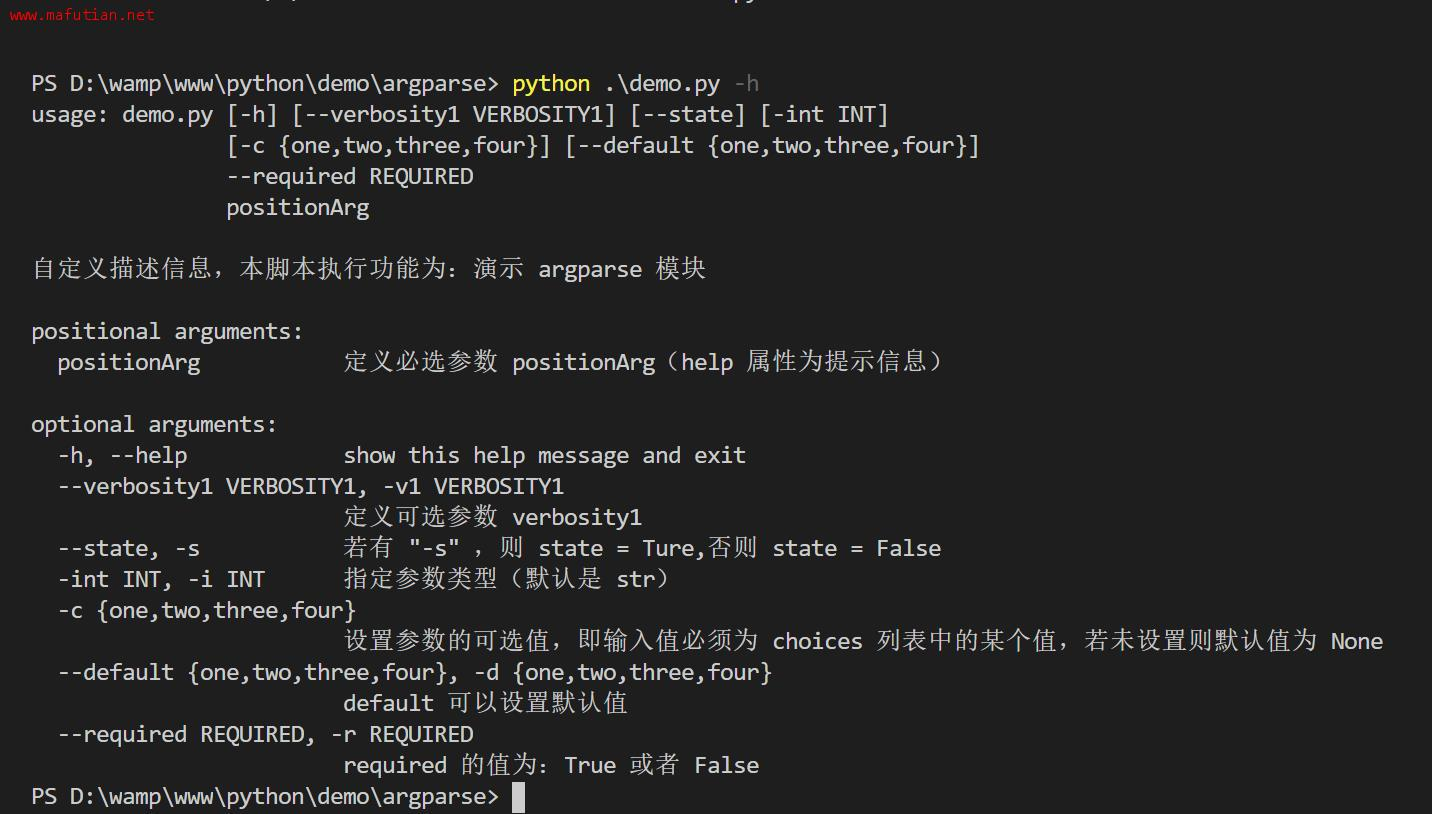2

0

 评论审核未开启 回复后邮件通知我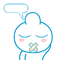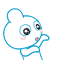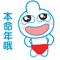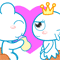• 浏览最多
• 评论最多